# ML Aggarwal Solutions for Class 7 Maths Chapter 7 Percentage and Its Applications

ML Aggarwal Solutions for Class 7 Maths Chapter 7 Percentage and Its Applications are available here. To cover the entire syllabus in Maths, the ML Aggarwal is an essential material as it offers a wide range of questions that test the students’ understanding of concepts. Students gain more knowledge by referring to ML Aggarwal Solutions for Class 7 Maths.

Chapter 7 – Percentage and Its Applications are available for download in pdf format. Percentage is a number or ratio expressed as a fraction of 100. It is often denoted using the percent sign, “%”. Suppose a student obtains 90 marks out of 100 in Maths, we can say that he has obtained 90 percent marks in Maths. Topics covered in this ML Aggarwal Solutions for Class 7 Maths Chapter 7 include convert a fraction into a percentage, convert a percentage into a fraction, percentage as a ratio, convert a given ratio into a percentage.

## Download the PDF of ML Aggarwal Solutions for Class 7 Maths Chapter 7 Percentage and Its Applications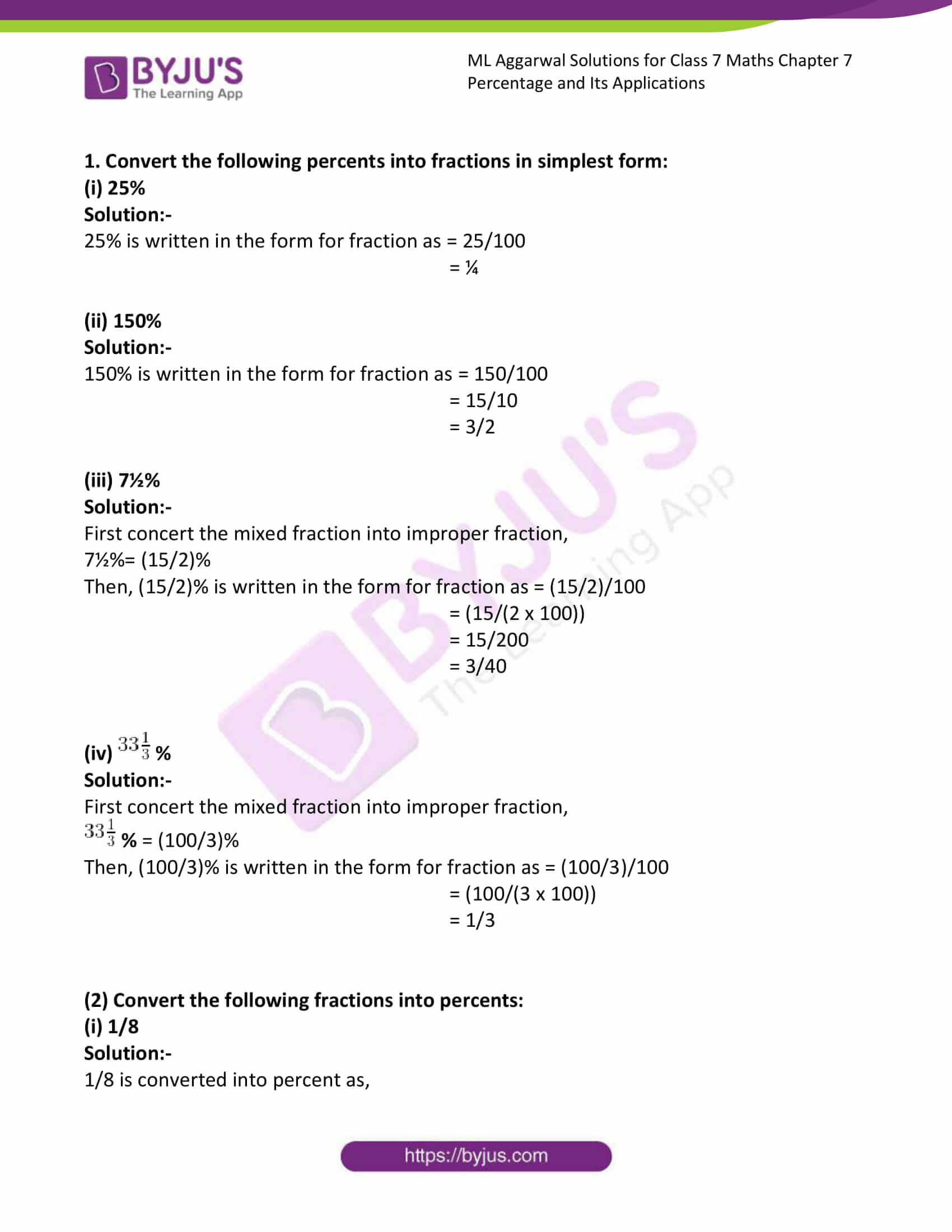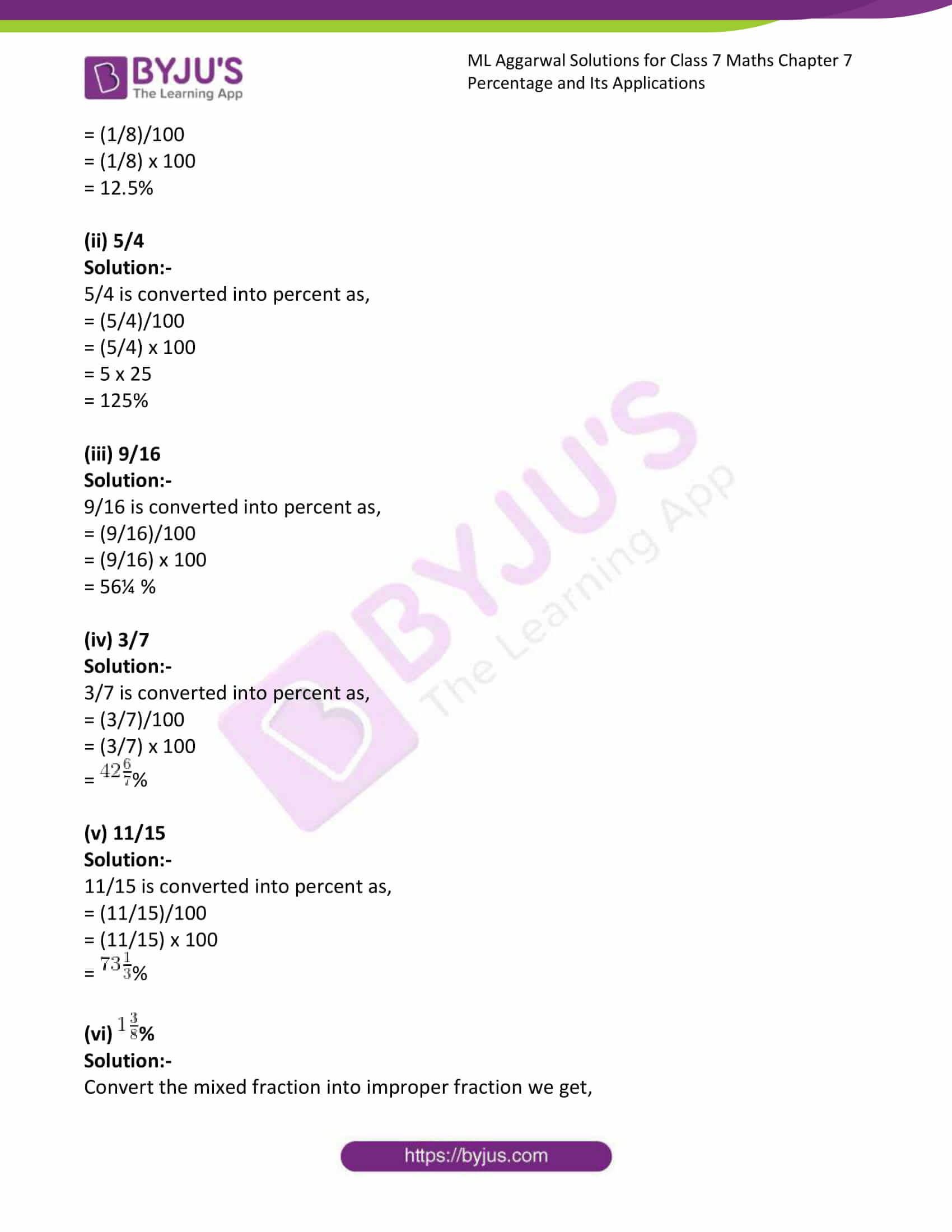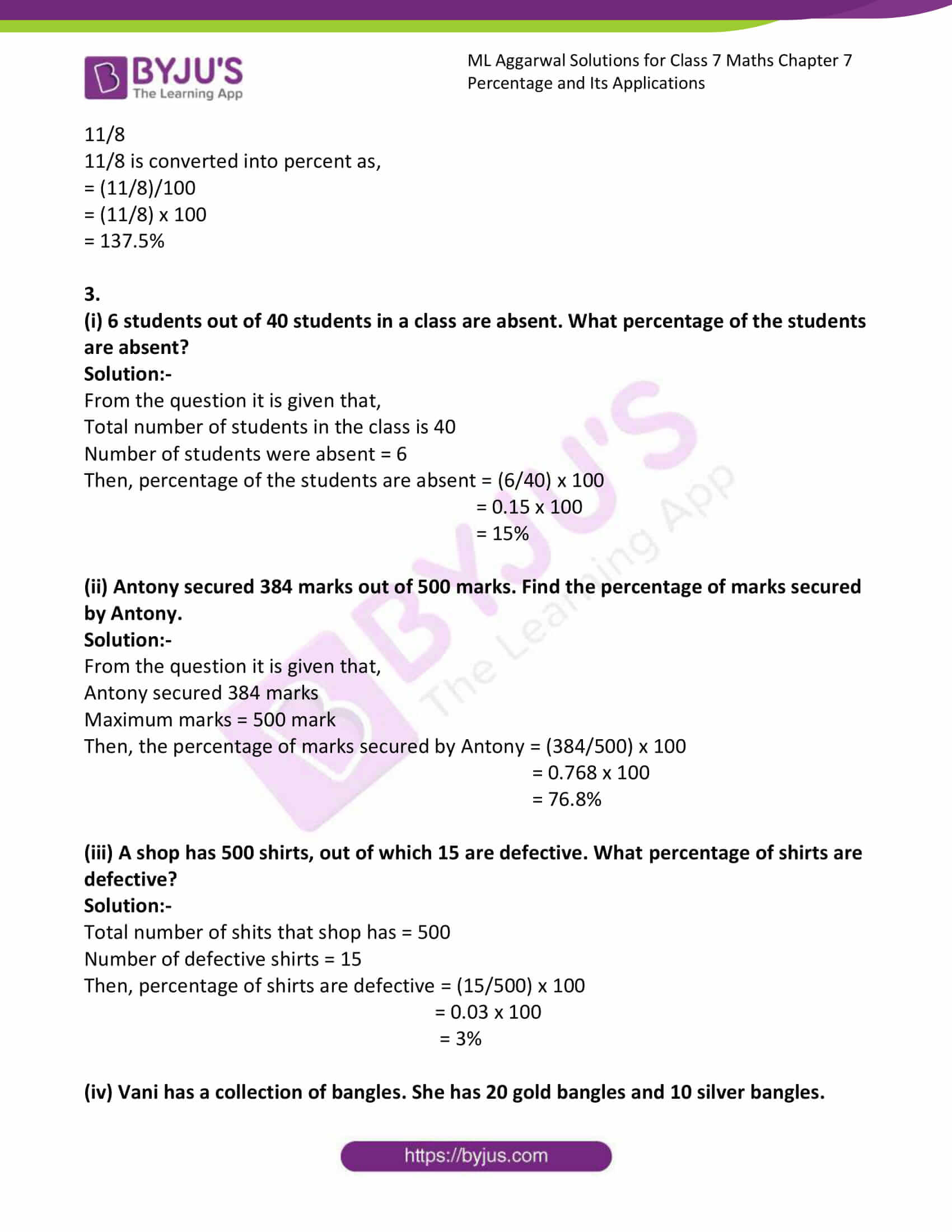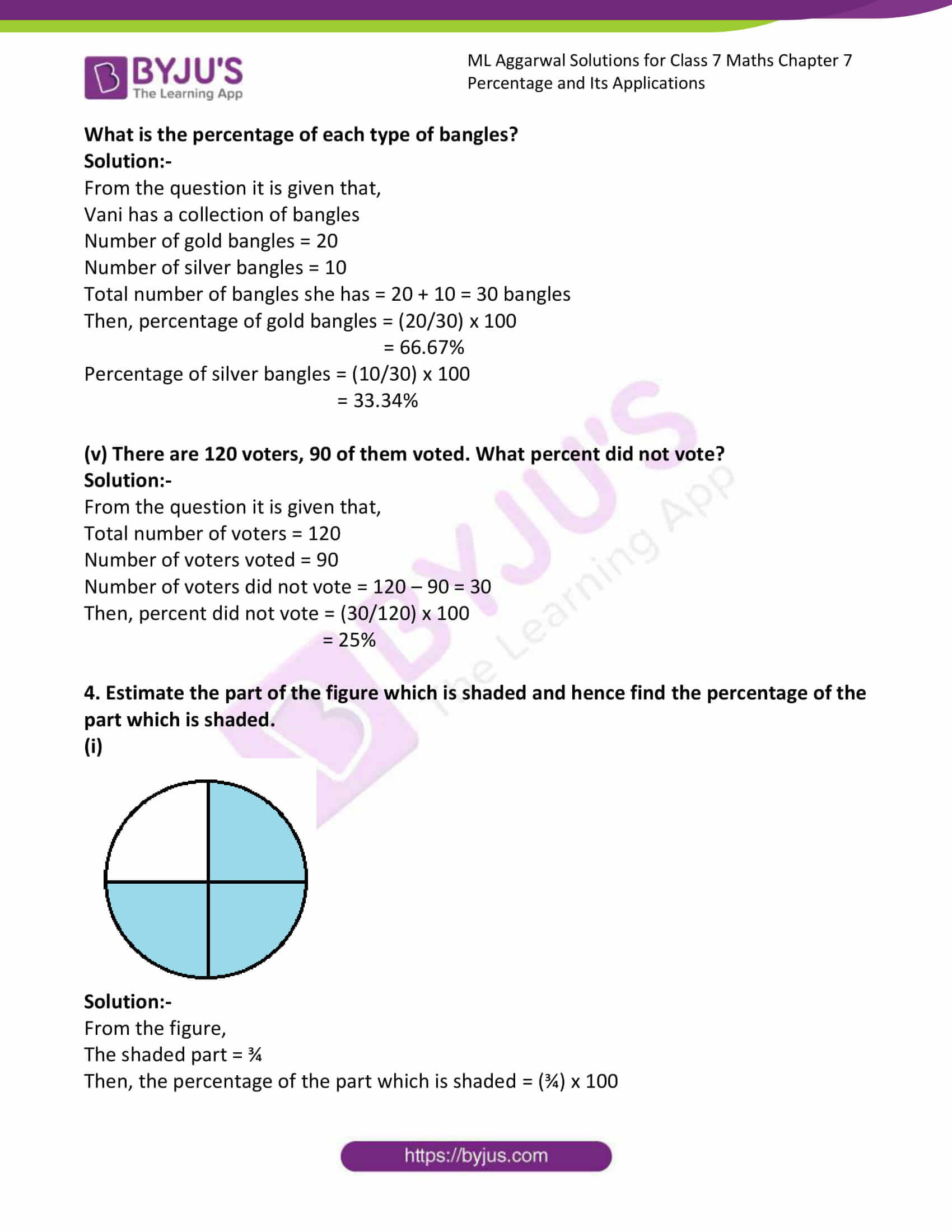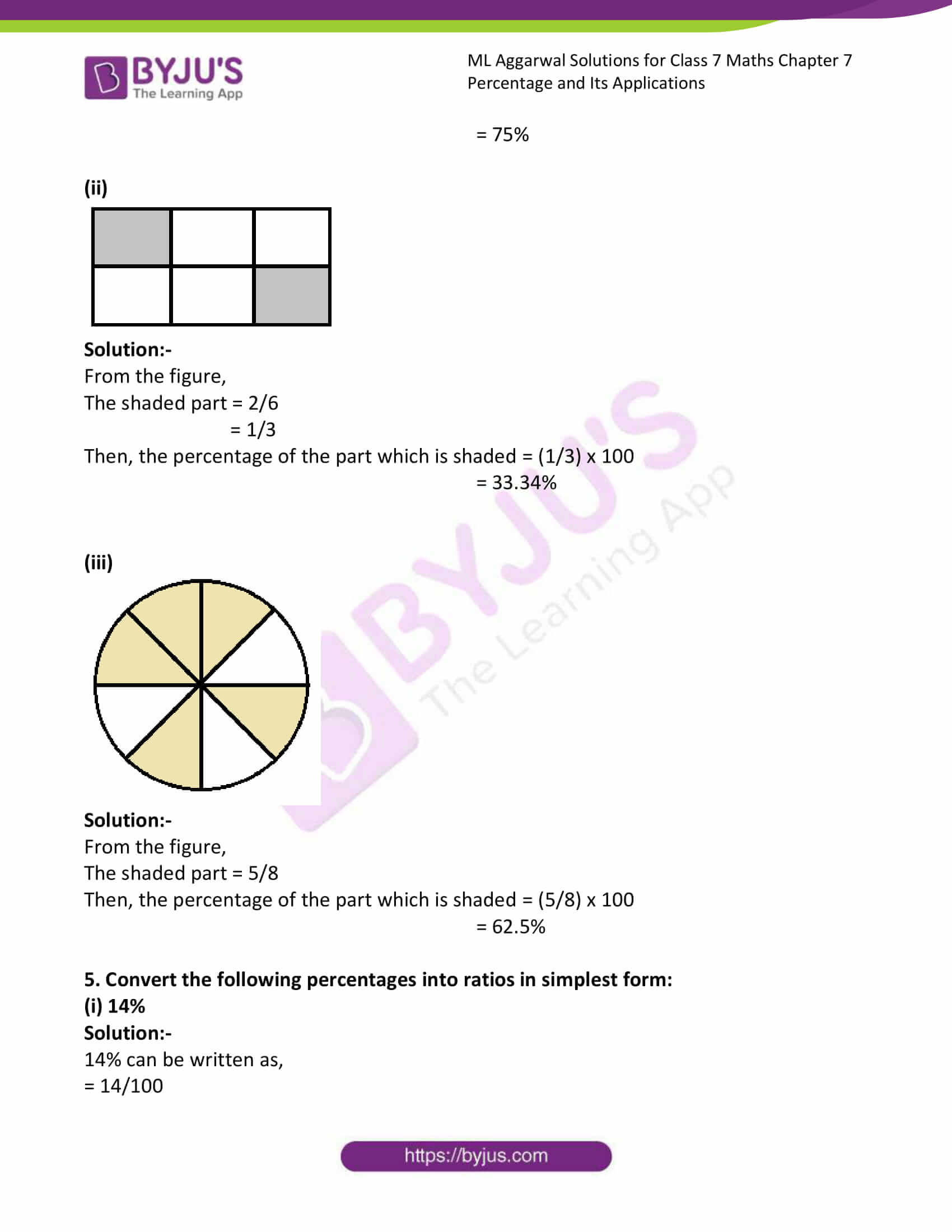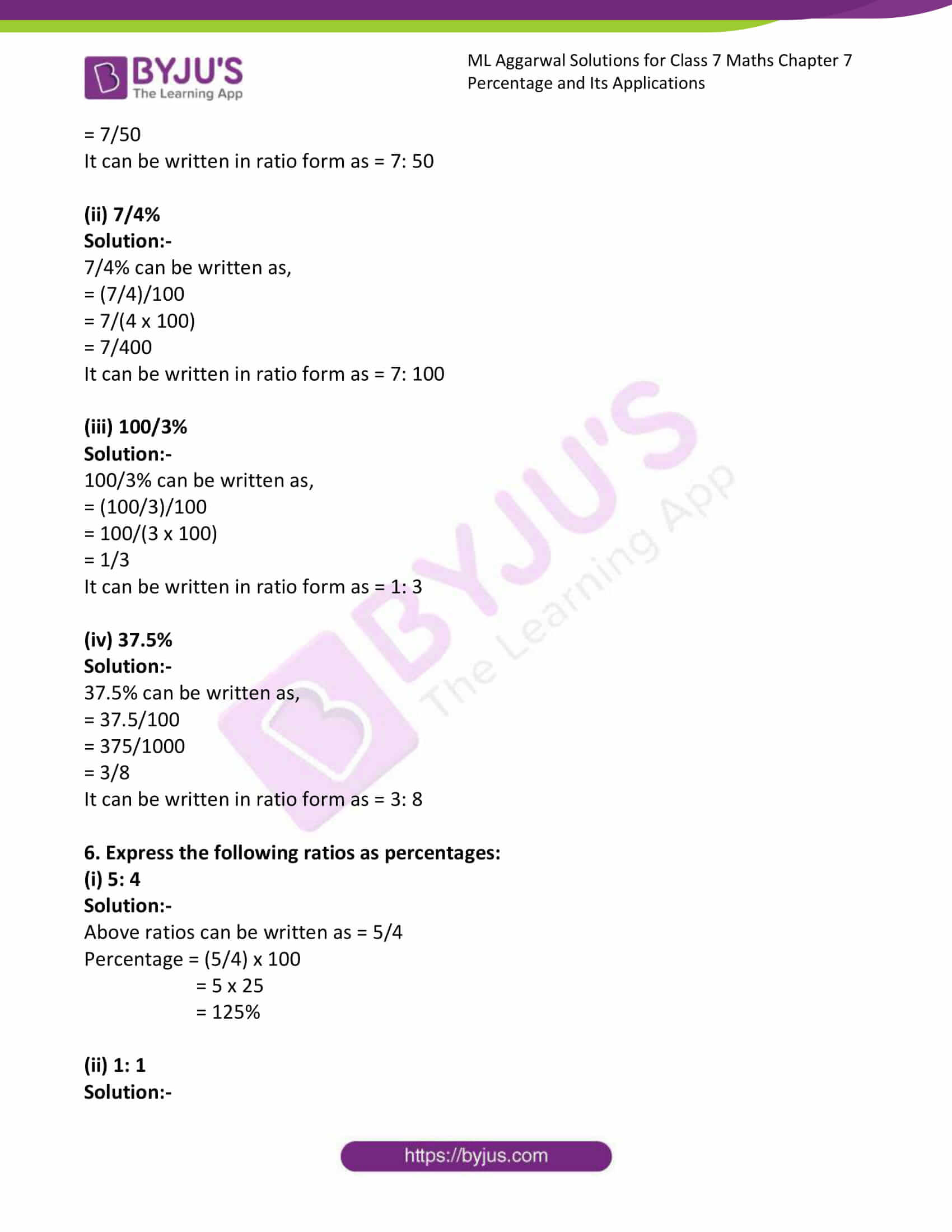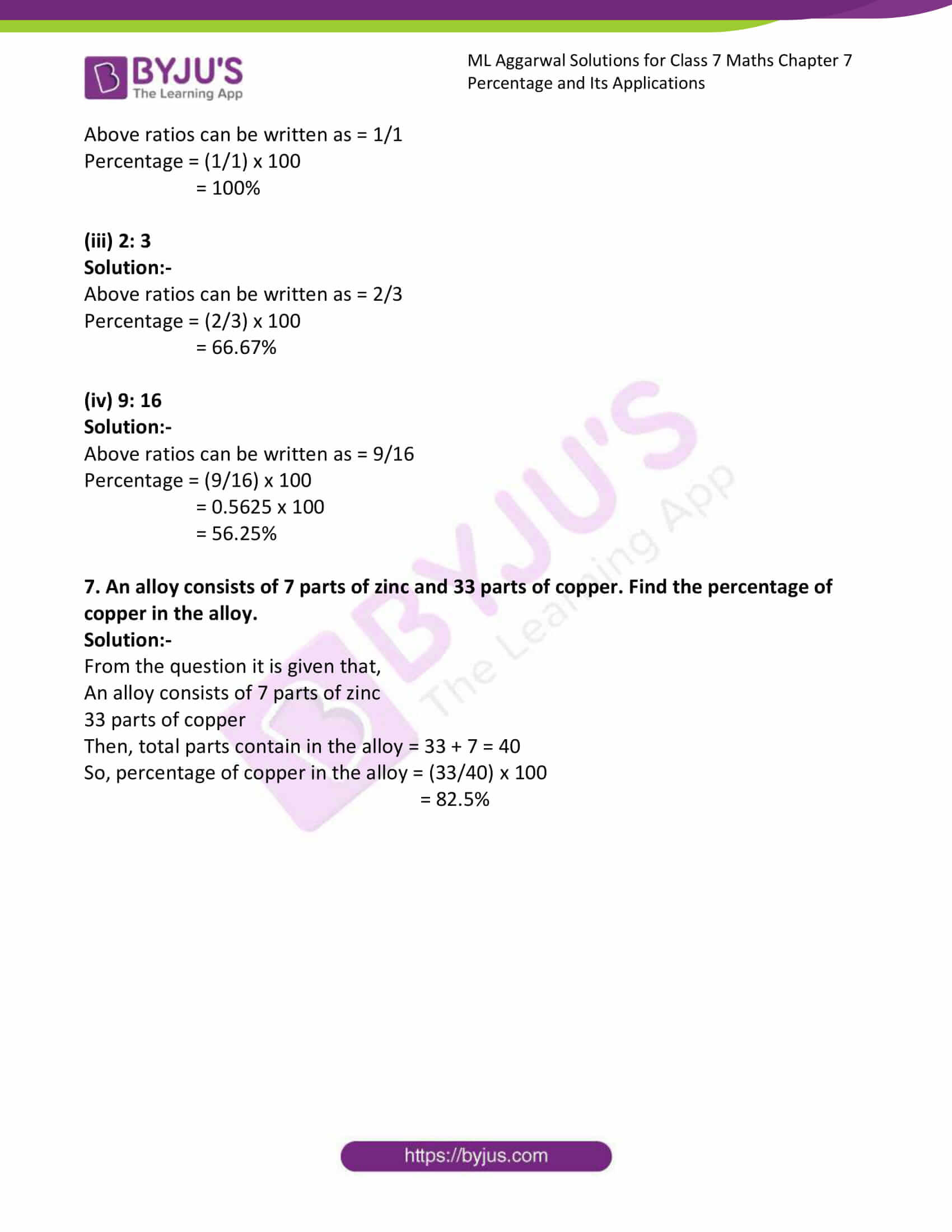### Access answers to ML Aggarwal Solutions for Class 7 Maths Chapter 7 Percentage and Its Applications

1. Convert the following percents into fractions in simplest form:

(i) 25%

Solution:-

25% is written in the form for fraction as = 25/100

= ¼

(ii) 150%

Solution:-

150% is written in the form for fraction as = 150/100

= 15/10

= 3/2

(iii) 7½%

Solution:-

First concert the mixed fraction into improper fraction,

7½%= (15/2)%

Then, (15/2)% is written in the form for fraction as = (15/2)/100

= (15/(2 x 100))

= 15/200

= 3/40

(iv)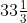%

Solution:-

First concert the mixed fraction into improper fraction,% = (100/3)%

Then, (100/3)% is written in the form for fraction as = (100/3)/100

= (100/(3 x 100))

= 1/3

(2) Convert the following fractions into percents:
(i) 1/8

Solution:-

1/8 is converted into percent as,

= (1/8)/100

= (1/8) x 100

= 12.5%

(ii) 5/4

Solution:-

5/4 is converted into percent as,

= (5/4)/100

= (5/4) x 100

= 5 x 25

= 125%

(iii) 9/16

Solution:-

9/16 is converted into percent as,

= (9/16)/100

= (9/16) x 100

= 56¼ %

(iv) 3/7

Solution:-

3/7 is converted into percent as,

= (3/7)/100

= (3/7) x 100

=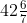%

(v) 11/15

Solution:-

11/15 is converted into percent as,

= (11/15)/100

= (11/15) x 100

=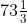%

(vi)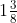%

Solution:-

Convert the mixed fraction into improper fraction we get,

11/8

11/8 is converted into percent as,

= (11/8)/100

= (11/8) x 100

= 137.5%

3.

(i) 6 students out of 40 students in a class are absent. What percentage of the students are absent?

Solution:-

From the question it is given that,

Total number of students in the class is 40

Number of students were absent = 6

Then, percentage of the students are absent = (6/40) x 100

= 0.15 x 100

= 15%

(ii) Antony secured 384 marks out of 500 marks. Find the percentage of marks secured by Antony.

Solution:-

From the question it is given that,

Antony secured 384 marks

Maximum marks = 500 mark

Then, the percentage of marks secured by Antony = (384/500) x 100

= 0.768 x 100

= 76.8%

(iii) A shop has 500 shirts, out of which 15 are defective. What percentage of shirts are defective?

Solution:-

Total number of shits that shop has = 500

Number of defective shirts = 15

Then, percentage of shirts are defective = (15/500) x 100

= 0.03 x 100

= 3%

(iv) Vani has a collection of bangles. She has 20 gold bangles and 10 silver bangles. What is the percentage of each type of bangles?

Solution:-

From the question it is given that,

Vani has a collection of bangles

Number of gold bangles = 20

Number of silver bangles = 10

Total number of bangles she has = 20 + 10 = 30 bangles

Then, percentage of gold bangles = (20/30) x 100

= 66.67%

Percentage of silver bangles = (10/30) x 100

= 33.34%

(v) There are 120 voters, 90 of them voted. What percent did not vote?

Solution:-

From the question it is given that,

Total number of voters = 120

Number of voters voted = 90

Number of voters did not vote = 120 – 90 = 30

Then, percent did not vote = (30/120) x 100

= 25%

4. Estimate the part of the figure which is shaded and hence find the percentage of the part which is shaded.

(i)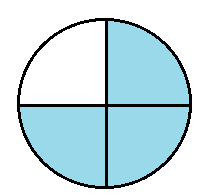Solution:-

From the figure,

Then, the percentage of the part which is shaded = (¾) x 100

= 75%

(ii)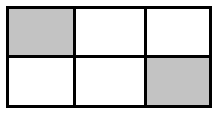Solution:-

From the figure,

= 1/3

Then, the percentage of the part which is shaded = (1/3) x 100

= 33.34%

(iii)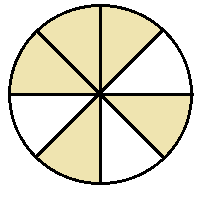Solution:-

From the figure,

Then, the percentage of the part which is shaded = (5/8) x 100

= 62.5%

5. Convert the following percentages into ratios in simplest form:

(i) 14%

Solution:-

14% can be written as,

= 14/100

= 7/50

It can be written in ratio form as = 7: 50

(ii) 7/4%

Solution:-

7/4% can be written as,

= (7/4)/100

= 7/(4 x 100)

= 7/400

It can be written in ratio form as = 7: 100

(iii) 100/3%

Solution:-

100/3% can be written as,

= (100/3)/100

= 100/(3 x 100)

= 1/3

It can be written in ratio form as = 1: 3

(iv) 37.5%

Solution:-

37.5% can be written as,

= 37.5/100

= 375/1000

= 3/8

It can be written in ratio form as = 3: 8

6. Express the following ratios as percentages:
(i) 5: 4

Solution:-

Above ratios can be written as = 5/4

Percentage = (5/4) x 100

= 5 x 25

= 125%

(ii) 1: 1

Solution:-

Above ratios can be written as = 1/1

Percentage = (1/1) x 100

= 100%

(iii) 2: 3

Solution:-

Above ratios can be written as = 2/3

Percentage = (2/3) x 100

= 66.67%

(iv) 9: 16

Solution:-

Above ratios can be written as = 9/16

Percentage = (9/16) x 100

= 0.5625 x 100

= 56.25%

7. An alloy consists of 7 parts of zinc and 33 parts of copper. Find the percentage of copper in the alloy.

Solution:-

From the question it is given that,

An alloy consists of 7 parts of zinc

33 parts of copper

Then, total parts contain in the alloy = 33 + 7 = 40

So, percentage of copper in the alloy = (33/40) x 100

= 82.5%